•感谢赞赏！给好友秀一下吧

内容棒，扫码分享给好友

•• 评论
• 收藏
•点赞点赞

# 谁是世界上最孤独的数？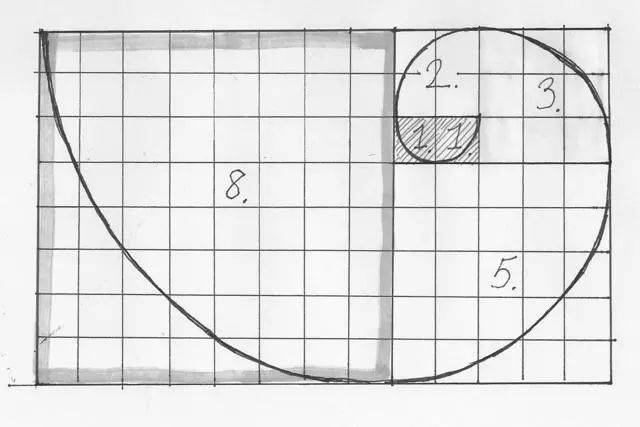• 第一级近似：7，于是它变成了 7 + 65/137。

• 第二级近似：把第一级留下的分数倒过来，137/65 近似是2，于是它变成了 2 + 7/65，于是开始的那个数字就变成了 7 + 1 / ( 2 + 7/65 )。

• 第三级近似：对7/65进行类似处理，以此类推。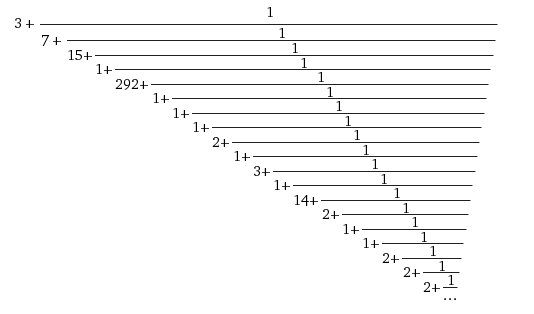π ≈ 3 + 1/7 = 22/7 ≈ 3.142...

π ≈ 3 + 1 / ( 7 + 1 / （15 + 1） ) = 3 + 1 / ( 113 / 16 ) = 355/113 ≈ 3.1415929...

| α - p/q | < 1 / q^2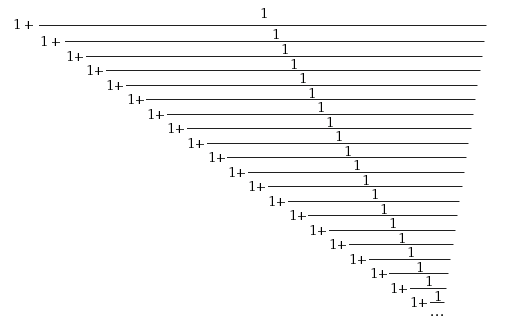2/1 = 2

3/2 = 1.5

5/3 = 1.66666...

8/5 = 1.6

13/8 = 1.625

21/13 = 1.61538...

（你可能注意到了，这个连分数的每一级逼近，就是传说中的斐波那契数列。为什么？你猜。）

1是最小的正整数。因此，φ，这个全部由1组成的连分数，是所有数中最难以接近的数。没有之一。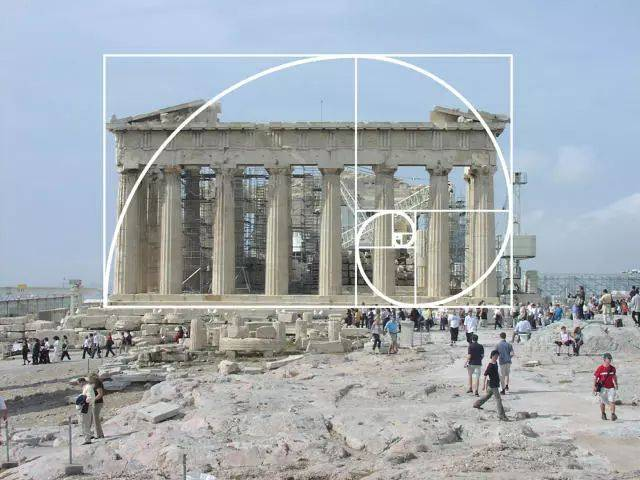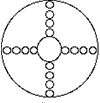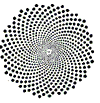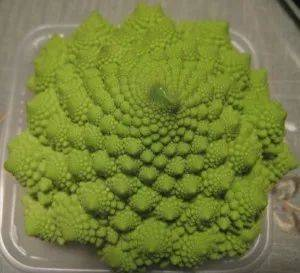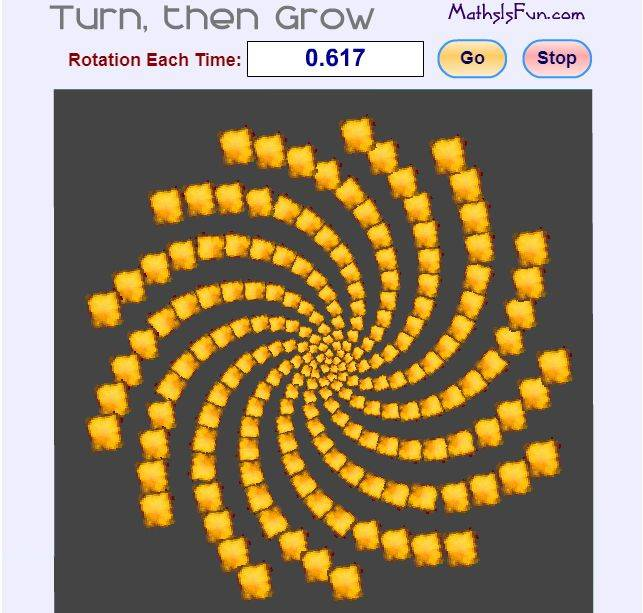*文章为作者独立观点，不代表虎嗅网立场

+1
49

0人已赞赏